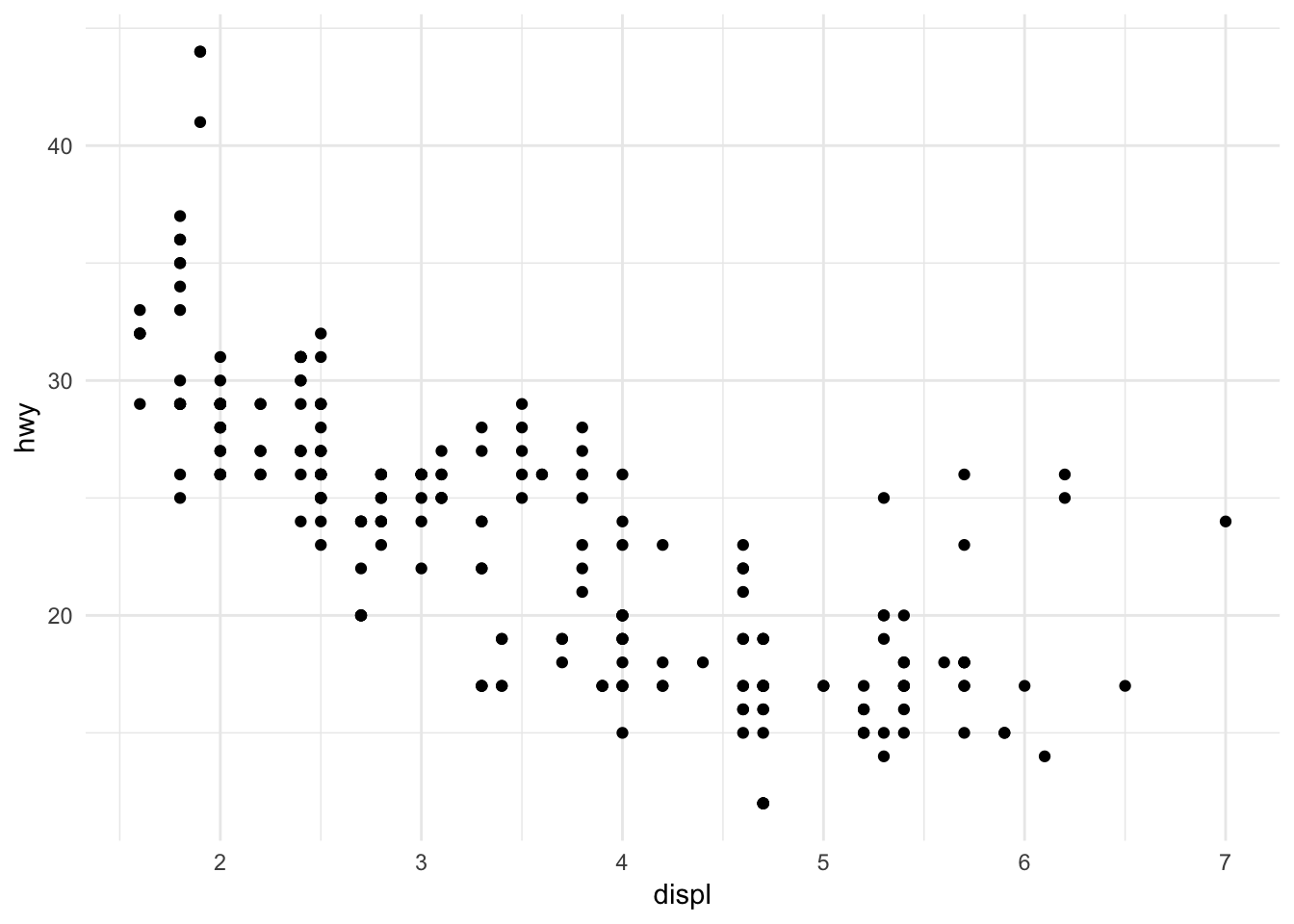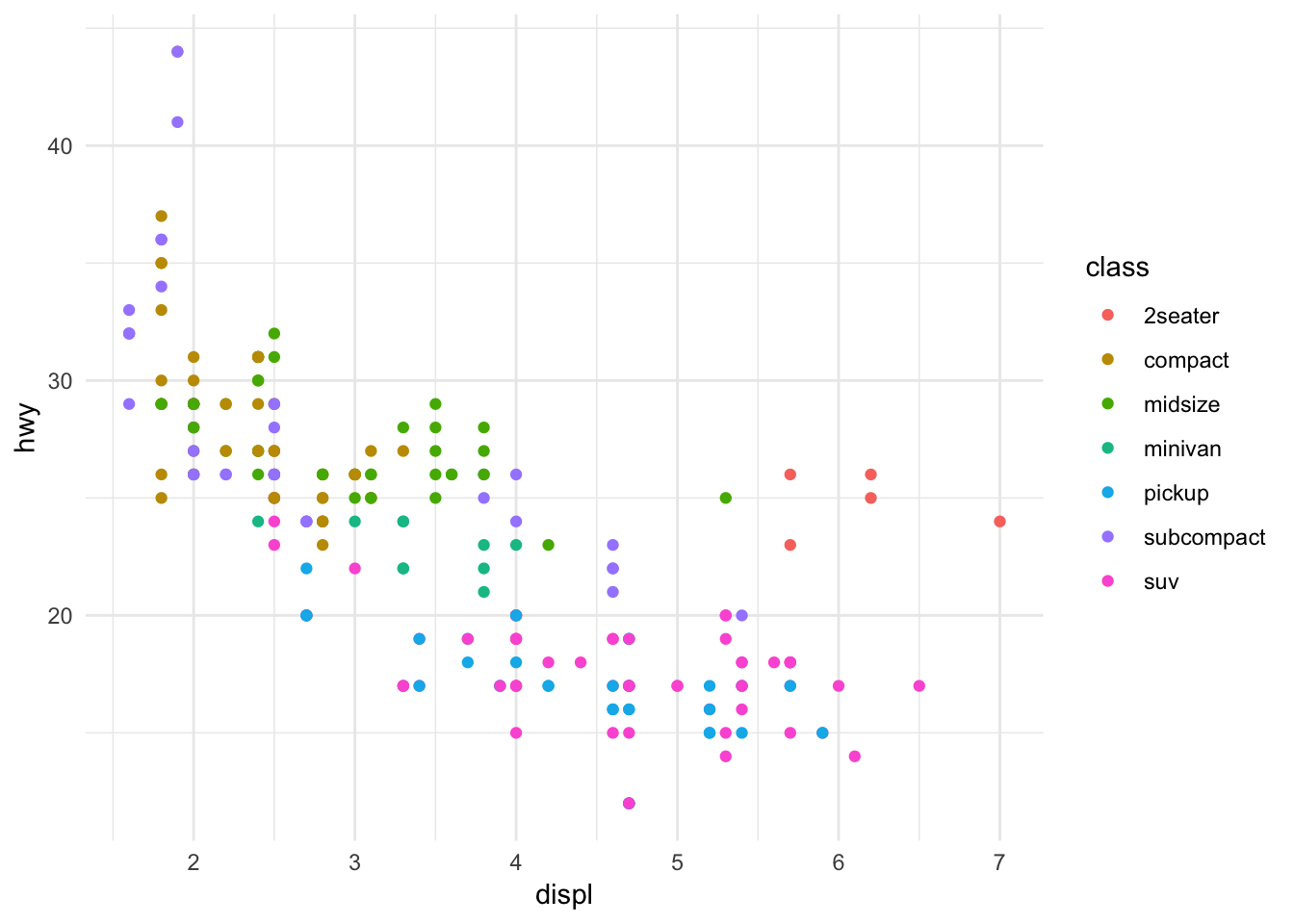## Miles Per Gallon

Make sure to start by running the setup chunk above to load the required packages. Then, read in the mpg dataset. There is one row for each particular type of car, with information about the fuel efficiency and other metadata.

``mpg <- read_csv("https://statsmaths.github.io/stat_data/mpg.csv")``

The variable `displ` gives the size of the engine in litres and `cty` the reported miles per gallon of the car while driving in stop-and-go city traffic. Likewise, `hwy` is the miles per gallon for a car on the highway.

Start by making a scatter plot (i.e., points) of engine size on the x-axis and the highway fuel efficiency on the y-axis.

``````ggplot(mpg, aes(displ, hwy)) +
geom_point()``````Verify that the pattern in this plot makes sense.

Next, modify the plot so that the color of the points show the class of the vehicle.

``````ggplot(mpg, aes(displ, hwy)) +
geom_point(aes(color = class))``````Identify a few different classes of vehicles and verify that they appear where you would expect on the plot.

Now, make all of the points in the plot twice as big and color them the color green.

``````ggplot(mpg, aes(displ, hwy)) +
geom_point(size = 2, color="olivedrab")``````